Identifying influential nodes based on graph signal processing in complex networks*
Department of Electronics and Information Engineering, Huazhong University of Science and technology, Wuhan 430074, China

Corresponding author. E-mail: hustlyu@hust.edu.cn

*Project supported by the National Natural Science Foundation of China (Grant No. 61231010) and the Fundamental Research Funds for the Central Universities, China (Grant No. HUST No. 2012QN076).

Abstract

Identifying influential nodes in complex networks is of both theoretical and practical importance. Existing methods identify influential nodes based on their positions in the network and assume that the nodes are homogeneous. However, node heterogeneity (i.e., different attributes such as interest, energy, age, and so on) ubiquitously exists and needs to be taken into consideration. In this paper, we conduct an investigation into node attributes and propose a graph signal processing based centrality (GSPC) method to identify influential nodes considering both the node attributes and the network topology. We first evaluate our GSPC method using two real-world datasets. The results show that our GSPC method effectively identifies influential nodes, which correspond well with the underlying ground truth. This is compatible to the previous eigenvector centrality and principal component centrality methods under circumstances where the nodes are homogeneous. In addition, spreading analysis shows that the GSPC method has a positive effect on the spreading dynamics.

Keyword: 89.75.–k; 02.30.Nw; 02.70.Hm; complex networks; graph signal processing; influential node identification
1. Introduction

Complex networks have attracted much attention in recent years since they meet the urgent need to understand the structures and dynamics of many real systems. In this research field, identifying influential nodes is an essential task. A small fraction of influential nodes can greatly affect the dynamics of networks, such as disease spreading,  information propagation, [4, 5] election,  and cascading failures. Moreover, the identification of the influential nodes plays an important role in controlling rumor spreading, defining new marketing strategies, and even predicting the total sale.[8, 9] All of these practical applications contribute to the importance of identifying influential nodes in complex networks.

In previous work, nodes occupying important positions in the network topology are regarded as important in complex networks. Known methods of ranking node centrality include degree centrality, closeness centrality,  betweenness centrality,  cluster centrality,  and eigenvector centrality. The degree centrality is straightforward and identifies the most connected nodes. The closeness centrality identifies those near to other nodes most. The betweenness centrality identifies those located on the most traveled paths. The cluster centrality identifies those with high clustering coefficient and influential neighbors. The eigenvector centrality (i.e., EVC, including PageRank and subsequent LeaderRank) considers nodes connected to other high degree nodes as highly central. Since influential nodes identified by the EVC tend to locate in a restricted region of the network topology, the principal component centrality (PCC) method using several principal eigenvalues is proposed as a global view.

The prior methods mainly focus on the network topology assuming that the nodes are homogeneous. However, in reality, node heterogeneity exists. For example, in wireless sensor networks, some nodes may be of different batteries to prolong their lifetime and reliability. Even homogeneous sensors have different levels of initial energy, depletion rate, etc. As a matter of fact, node heterogeneity greatly affects routing, epidemic spread, and safety deployment. Besides, the topological equivalence of homogeneous networks (e.g., regular networks, small-world networks) makes the network topology based node centrality approximately equal. Therefore, it is necessary to adopt node attributes as a supplement for ranking node centrality. Moreover, owing to the accumulated prior knowledge of complex networks, node attributes entitle us to rank the node centrality more comprehensively. In other words, node heterogeneity needs to be taken into account in the influential node identification.

In this paper, we propose a graph signal processing based centrality (GSPC) method to identify the influential nodes considering both the node heterogeneity and the network topology. The first intuitive step is to derive an overall evaluation of node heterogeneity (i.e., different attributes such as interest, energy, age, and so on), which is called the node signal. We categorise node attributes into three types and propose a modulated 2-norm based model to obtain the node signal. In order to integrate the node signals with the network topology, we apply the graph signal processing method. In particular, the node signals are processed by the graph Fourier transform. Signal processing techniques and methodologies are used to pick up the most principal eigenvectors. Then, the node centrality is calculated by the combination of the node signal and the selected eigenvectors. By this means, we identify the influential nodes with both node attributes and the network topology.

We first evaluate our proposed method in two real-world datasets with node heterogeneity information and underlying ground truth. We have three major findings. First, identified nodes based on our GSPC method corresponds well with the underlying ground truth. Second, GSPC degenerates to the previous EVC and PCC methods under the circumstance where the nodes are homogeneous. Furthermore, the results under varying parameters and null models prove the stability of our GSPC method. In addition, spreading in a synthetic network and a real social network is investigated, which indicates that the GSPC method has a positive effect on the spreading dynamic.

The rest of this paper is organized as follows. Section  2 presents the modulated 2-norm based evaluation model of node heterogeneity. In Section  3, we propose a GSPC method which integrates both node attributes and the network topology. The data description and centrality measures of two networks are, respectively, given in Subsections  4.1 and 4.2, compatibility analysis and stability analysis are, respectively, discussed in Subsections  4.3 and 4.4. Then, spreading is analyzed in Subsection  4.5. Section  5 ends this paper with some conclusions.

2. Evaluation of node heterogeneity

Node behaviors are significantly affected by node heterogeneity (i.e., different node attributes) in various networks. For example, individuals in social networks determine their interactions concerning age, gender, profession, opinions, and so on, while nodes in wireless networks forward packets due to trust, delay, battery, and so on. Therefore, in this section, node attributes are investigated and a modulated 2-norm based model is proposed to achieve an overall evaluation of each node.

We divide node attributes into three types. Firstly, since dynamics like rumor control, immunization, and opinion diffusion involve opposite sides, parts of the node attributes are used to decide the sign, which is denoted as g. The sign type refers to whether or not you could support, ignore, or oppose an event, which can be modeled as a triple g ∈ (+ 1, 0, − 1), where + 1, 0, − 1 stand for endorsement, disinterest, and opposition, respectively. For example, for opinions diffusion in social networks, if node i stands for one opinion, g is positive. And if the node is opposed to the opinion, it should be negative. Otherwise, if it pays no attention, g = 0, that is, it is insignificant in the network dynamics.

Besides, analogous to the trust evaluation of nodes,  each node has two other types of node attributes: confidence and ability. The confidence type corresponds to the probability at which nodes function like online time, activity, and frequency of tweets, denoted as t. For a simplified example, the online time in social networks could be modeled as a probabilistic model. For a specific hour in a day, if a node gets online α times in β days, then the probability that this node gets online in this hour is t = α /β according to the minimum unbiased estimation.

The ability type corresponds to the potential service provided by the nodes, denoted as a. A high ability means that the node has more potential to respond to dynamics on the networks fast and wide. Owing to the development of computer science, empirical analysis[9, 22] of various social networks have sprung up. Empirical data of a tweet indicates that a user generally follows experts on various topics of her/his interest. Elite users like media, celebrities, bloggers, and organizations roughly are 0.05% of the population and account of about 50% attention.[24, 25] Other personal information like age, gender, location, and so on is less mentioned. Thus identity (e.g., celebrities, actors, writers), role (e.g., connectors, experts, and salesman) and interest (e.g., favorite book, music, and so on) are regarded as some of the more important node attributes in social networks. In this way, we roughly classify the ability attributes into two categories in Table  1.Table 1. The empirical classification of the ability attributes.

Because ability attributes have different importances, coefficients η i are introduced to modulate the significance of each attribute ai based on two rules. First, make sure that η 1 > η 2 if a1 belongs to the important category while a2 belongs to the less important category. Attributes that belong to the same category are assigned the same coefficients. Second, ∑ iη i = 1. Examples and details of η will be discussed in the stability analysis in Subsection  4.4.

Then, node ability is codified as the distance to the zero point and is calculated by a modulated 2-norm model. That is, a = ‖ η a2 = |η 1a1+ · · · + η NaN| (∑ iη i = 1, N is the number of the ability attributes). Without loss of generality, we just take 3-dimension as a simple example, as shown in Fig.  1.

 Figure Option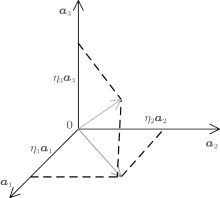Fig.  1. A simple example of nodes with three ability attributes. It is codified as a distance to the zero point. And each attribute is modulated according to its importance.

In conclusion, the overall evaluation of node i is calculated as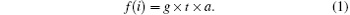It could be either positive or negative, the higher the absolute value is, the more important role the node plays in networks.

With an overall evaluation of node attributes at each node, denoted as the node signal, a complex network can be intuitively represented as a graph with node signals on it, as in the example of the Zachary club network shown in Fig.  2. Each node has a value f (i). The blue line means positive, and the red line means negative. The height represents the absolute value of each node. Nodes 10, 17, and 19 have zero signal because they do not support either node 1 or node 34 in the Zachary club network. By this means, we can integrate node heterogeneity with the network topology to identify influential nodes in the next section.

 Figure Option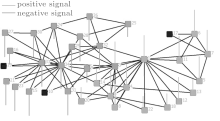Fig.  2. Example of the Zachary club network. After an overall evaluation of each node, the Zachary club network can be represented as a graph with node signals on it. There is no signal on the black nodes. Red means negative signal, while blue means positive signal. The height is the absolute value of each signal.

3. Graph signal processing centrality (GSPC) method

In order to unite node attributes with the network topology together to identify influential nodes, we propose a graph signal processing centrality method in this section.

Signal processing on graphs focuses on the interplay between the graph topology and the graph signals. For signal processing on graphs, a spectral graph theory has been explored as a tool to define expansion bases for graph Fourier transforms. Many important mathematical ideas and intuitions can be extended from the classical Fourier analysis to the graph setting. By this means, main bases are picked up to do compression, filter, and so on.

Let A denote the adjacency matrix of a network with N vertexes, E edges, and a node evaluation set f, graph G = (N, E, f). When a link is presented between two nodes vi and vj, both Ai, j and Aj, i are set to 1, otherwise they are set to 0. Since A is a real symmetric matrix and diagonalizable, it has a complete set of orthogonal eigenvectors denoted by ul (l = 1, … , N) and corresponding eigenvalues λ l (l = 1, … , N, λ 1λ 2 ≤ · · · λ N), which satisfy Aul = λ lul.

According to the classic Fourier transform, it turns the time domain signal into the frequency domain signals, which turns complex things into independent components. That is,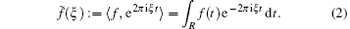According to the spectrum, the main frequency band is reserved by an appropriate filter. Thus, the main component of the spectrum is picked up, which could be used to reconstruct the original signal f (t).

Analogously, treating the evaluation of node attributes as signals, the graph Fourier transform is utilized to find the principal eigenvectors of the adjacency matrix A. Since the eigenvectors of the adjacency matrix are used to measure the topology importance of the nodes, [1416, 28] we define the graph Fourier transform to identify influential nodes as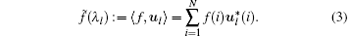This graph Fourier transform gives us a way to represent both the network topology and the node attributes in the graph spectral domain. According the the Fourier analysis, the signals are compressible when the graph Fourier coefficients decay rapidly. If it is compressible, then several principal eigenvectors selected according to the signals in the spectral domain are used to represent the network under the node heterogeneity.

Thus, according to the spectrum, p appropriate eigenvectors are selected to represent the main information of the network topology. Choosing an appropriate p is important. We choose p analogous to that in the EVC or PCC method. The first idea is similar to the EVC method, we choose the eigenvector with the highest spectral value, that is,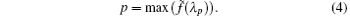This is suitable for the case where one spectral value is much more higher than the others. Otherwise, we list spectral values in descending order and then choose p according to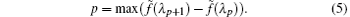That is, if the next value (λ p+ 1) is much less than current one (λ p), then the spectral values after the p-th would be ignored, and so are the corresponding eigenvectors. In this way, the principle eigenvectors are picked up.

Combing the node heterogeneity, the centrality of the nodes is calculated as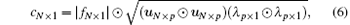where ⊙ is the Hadamard operator.

Equation  (6) states that the node centrality is determined by both the node signals and the network topology. The larger the node signal is, the higher the node centrality is. The more important the network position a node occupies, the higher the node centrality is.

We now analyz the time complexity. The GSPC method contains four parts step by step, that is, eigenvalues and eigenvectors calculation (O(N2)), Fourier transform (O(N log N)), selection (O(N log N)), and centrality computing (O(pN), where p is the number of selected eigenvectors and pN). Then, the time complexity of the GSPC method is O(N2).

The time complexity of the most simple degree centrality is O(E). For the most popular PageRank algorithm, its time complexity is O(EL), where L stands for the iterations. The time complexity for the betweenness centrality is O(NE). The time complexity of the recently proposed PCC algorithm is O(N2log2N) or O(N2log3N) to different networks topologies and PCC could be used in large-scale networks like Facebook data with 3097165 users. The time complexity of the GSPC method is higher than the former two and lower than the latter two.

4. Simulation and discussion

In this section, we first identify influential nodes by our GSPC method in two small real networks with ground truth. These networks contain not only the network topology information but also the node attributes information. Then the compatibility and stability of our GSPC method are analyzed. At last, spreading analysis is implemented both in a larger synthetic network and a larger real social network.

4.1. Zachary club network

The Zachary club network is a social network of friendships among 34 members of a karate club at a US university in the 1970s. During the observation period, for the disagreement between the administrator and instructor of the club, the club splits into two groups and one group leaves to start their own club. Mapping the network to the topological graph, the administrator and the instructor are represented by vertices 1 and 34, respectively.

Figure  3(a) shows the well known Zachary club network. It shows that two groups surround their own centers, nodes 1 and 34, respectively, and it has a very clearly divided community structure.

 Figure Option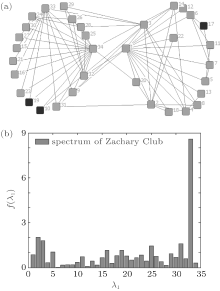Fig.  3. (a) The network topology and (b) spectrum of the Zachary club network.

First, we obtain the node signal as follows. As shown in Table  2, the support attribute belongs to the sign category and determines the sign to be negative or positive. In this case, nodes are set as + 1, − 1, and 0 corresponding to their support on nodes 1, 34, and none, respectively. The strength attribute belongs to the ability category and determines the degree of support. Thus, the node evaluation is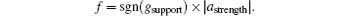By the graph Fourier transform, we calculate (λ l) and obtain the spectrum in Fig.  3(b). Given that the spectral value at λ 33 is much higher than the rest, we set p = 1. Then we pick up λ 33 and its corresponding eigenvector to rank the node centrality as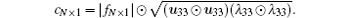Table 2. Node attributes, the corresponding node evaluation and comparison among the network topology based centrality methods and our GSPC method of the Zachary club network.

The results in Table  2 show that the node centrality obtained by the GSPC method conforms to the real situation of the Zachary club network because the centrality of nodes 1 and 34 are the two highest. In addition, we can find out that the network topology based methods distinguish nodes 1 and 34, which may be due to the Zachary karate club displaying a divided community structure and hence a distinct topology.

4.2. Krack-High-Tec managers network

The Krack-High-Tec managers network was collected from the managers of a high-tec company on the west coast of the United States. It has just over 100 employees with 21 managers. Mapping to topological graph, it has 21 nodes and the most important is node 7.

The topology is shown in Fig.  4(a) in which the nodes are too mixed with each other to tell the central node intuitively.

 Figure Option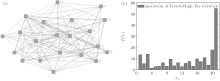Fig.  4. (a) Krack-High-Tec network topology and (b) spectrum.

Table  3 involves four attributes of the managers: age (in years), tenure (length of time employed by the company, in years), level in corporate hierarchy (coded 1, 2, 3), department of the company (coded 0, 1, 2, 3, 4). These attributes all belong to the ability category and we use the modulated 2-norm model to obtain the node signal.

In particular, age and department attributes are not in accordance with the tenure and level attributes (contribute to role of an individual a lot), so their coefficients are set rather small. For the level attribute, the smaller, the better, we turn the values of level upside down, that is, alevel = 4− alevel. Then the node signal is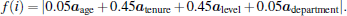Then by the graph Fourier transform, we obtain the spectrum in Fig.  4(b). According to Fig.  4(b), the spectral value at λ 21 exceeds the rest by a lot, thus p = 1 and λ 21, u21 is picked up. The node centrality is calculated as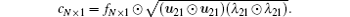In this way, we obtain the node centrality of the Krack-High-Tec network shown in Table  3. It shows that the node centrality obtained by our GSPC method conforms the ground truth of the Krack-High-Tec managers because the centrality of node 7 is the highest. For network topology based methods, the centrality of node 15 is the highest, which suggests that node 15 is the most important person in the network and turns out to be false. This indicates that the node attributes are dispensable in identifying influential nodes in complex networks.Table 3. Node attributes and comparison among the network topology based centrality methods and our GSPC method of the Krack-High-Tec network.
4.3. Compatibility analysis

We first test the compatibility of our GSPC method to the eigenvector based centrality methods such as the PCC and EVC methods. Assume that the nodes are all homogeneous and the node signals are all set to be 1. Then, our GSPC method is degenerated to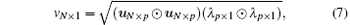which is of the same form as that in the PCC method. And when p = 1, PCC equals a scaled version of EVC. This indicates that our GSPC method is compatible to both the PCC and EVC methods under different p selection and could be effective with only topological information.

The GPSC method under homogenous nodes is used to calculate the node centrality in the above two networks, which is then compared to the PCC and EVC methods. The parameter r1 stands for the correlation coefficient, if it is more closer to 1, then the variables are more correlated; and r2 for testing the hypothesis of no correlation, the smaller the better.

Table  4 shows the correlation of our GSPC with the PCC and EVC in the Zachary club network. Since we chose p = 1 in our GSPC method, the result equals to that of the EVC method and is highly correlated to that of the PCC method. We also find that the top five nodes are the same, 1, 2, 3, 33, 34.

Similar to Table  4, Table  5 shows that our method is highly correlated with the PCC and EVC methods in the Krack-High-Tec network. We then find that the top five nodes are the same too, 2, 3, 15, 18, 20. However, the most important node 7 is not spotted, which suggests that the node attributes do affect the node centrality and are dispensable for the node centrality ranking.Table 4. The correlation of our GSPC method with the PCC and EVC methods in the Zachary club network.Table 5. The correlation of our GSPC method with the PCC and EVC methods in the Krack-High-Tec network.
4.4. Stability analysis

This subsection is mainly divided into two parts. First, we analyze the modulation of parameters of our GSPC model. Second, we analyze the stability of the topology and node signals.

In the first place, we vary the value of η to test the stability of our method satisfying the two rules aforementioned in Section  2. The test group includes [0.05, 0.45, 0.45, 0.05], [0.1, 0.4, 0.4, 0.1], and [0.2, 0.3, 0.3, 0.2] in the Krack-High-Tec network. It turns out that as long asη for the important attributes are larger than the others, we can still maintain the same node centrality ranking order and correctly find the influential nodes.

We test the effect of p on the node centrality ranking. The results in Table  6 and Table  7 show that when p varies from 1 to 3, both in the Zachary club network and the Krack-High-Tec network, the influential nodes are picked out in accordance to the ground truth.

Then, we analyze the stability of topology and node signals. For a null model, we randomly shuffle the edges while conserving the degree distribution. We then shuffle the node signals. The results are shown in Tables  6 and 7, respectively.Table 6. The results under varying p values and null models of our GSPC method in the Zachary club network.Table 7. The results under varying p values and null models of our GSPC method in the Krack-High-Tec network.

For the Zachary club network, the most influential nodes always change in both conditions. As in both cases, the node signals in one community are of different signs. The sign of the node signal greatly affects the node centrality ranking.

For the Krack-High-Tec network, we find that the node attributes play a more important role in node centrality ranking. When we shuffle the edges randomly, we still identify node 7 to be the most influential, and when we shuffle the attributes randomly, the node with the attributes of original node 7 is found to be the most influential.

In other words, it indicates that both network topology and node heterogeneity play an important role in influential node identification. In networks with a divided community, like the Zachary club network, the sign attributes are essential because they chaos the network topology so that neighbor nodes may be opponents instead of supporters. In networks with homogeneous topology, like the Krack-High-Tec network, the node heterogeneity becomes much more important in influential node identification.

As the previous works[12, 15] have pointed out, the influential nodes are verified by their efficiency in dynamics like spreading. That is, the nodes are taken as sources and their corresponding spreading efficiency is used to measure their influential importance. We apply the SI model[31, 32] to test the spreading efficiency.

In the SI model, the nodes are classified into two states, infected or suspectable. At the beginning, some nodes are chosen as the source and in the infected state while all others are in the susceptible state. The source nodes will spread to all her neighbors. In every time step and for every link connected to the infected nodes, the suspectable nodes become infected at a fixed probability λ .

We use two datasets in this section. The first one is a synthetic network developed by a BA model with gradual aging. The BA model captures the essential scale-free property of real networks by growth and preferential attachment. Since nodes have a finite lifetime or a finite capacity,  the probability a new node connecting to an old one is not only proportional to its degree but also depends on its age, decaying as τ γ , γ < 1. Thus, with age decaying considered, the node signal is calculated as τ γ , γ = 0.5.

We then apply a real social network, a Facebook like forum network which originates from an online community for students at the University of California, Irvine. The dataset includes 899 users and the numbers of messages posted by the users. Then, the number of posted messages is referred to as the node signal.

By using the methods aforementioned and our GSPC method, we obtain the rank of nodes. Then several influential nodes are chosen as the source nodes for the SI model.

Figure  5 shows that in both cases, nodes spread faster where the sources are the most influential nodes chosen by our GSPC method compared to the other methods. As shown in Fig.  5(a), the GSPC method outperforms the other methods because it adds node attributes into consideration. The closeness and PCC methods gain a better performance than the other three. Since the BA model based networks have small cluster coefficients, the influential nodes obtained by the closeness method are scattered. The PCC methods identify influential nodes at a global view. The EVC method performs the worst, as the influential nodes under the EVC method gather in one restricted region of the network topology.

 Figure Option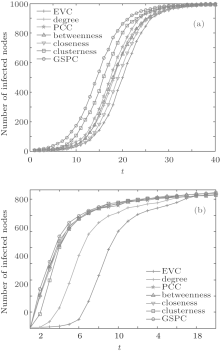Fig.  5. SI spreading in a BA model based network and the Facebook like forum network. The results are the average over 200 independent realizations: (a) network size N = 1000, λ = 0.3; (b) network size N = 899, λ = 0.3.

As shown in Fig.  5(b), the GSPC method performs well in spreading. It is applicable in real social networks and indicates that the top five influential nodes are identified effectively. The EVC method results in bad performance, as the influential nodes gather in one restricted region of the network topology, which is the same as that in Fig.  5(a). Due to the positive correlation between the number of posted messages and the network topology in the Facebook like forum network, the influential nodes identified by PCC, betweenness, closeness, and cluster centrality are close to those by the GSPC method.

In summary, the GSPC method has a positive role in spreading. It gains the insight of balance between the network topology and the node heterogeneity. Thus, it is very useful in network dynamics where both the network topology and the node heterogeneity matter.

5. Conclusion

Identifying influential nodes for complex networks is an important task. A graph signal processing based centrality method is proposed in this paper, which combines the network topology and the node attributes together to distinguish the influential nodes. First a modulated 2-norm model is presented to obtain an overall evaluation of the node attributes. Then, the graph Fourier transform is utilized to select principal eigenvectors. Together, the node centrality is calculated with both the network topology and the node attributes. The results show that the GSPC method is effective in influential node identification and it is compatible to the previous eigenvector based centrality methods, the PCC and EVC methods. In addition, we verify the stability of the GSPC method by varying parameters and null models. Also, the spreading performance is upgraded.

Our GSPC method takes node heterogeneity into account and extends the scope of the node centrality calculation. This paper shows that our method can identify influential nodes in signed graphs (the Zachary club network). There are some possible applications. For example, many real complex networks can be regarded as weighted networks. Ranking node centrality in weighted networks attracts lots of interest. By converting the weights on edges into the graph signal or use the weight matrix other than the adjacency matrix for the graph Fourier transform, our method is easy to transplant to weighted networks. For many real networks, nodes with constraints (for example, limited online time in social networks, node with limited capacity in power grid) have a strong impact on the node behaviors. Our GSPC method could explore these constraints and calculate the node centrality combining the node properties with the network topology.

Reference
 1 Barabási A L 2007 IEEE Control System Magazine 27 33 DOI:10.1109/MCS.2007.384127 [Cited within:1] [JCR: 2.372] 2 Yeung C H and Saad D 2013 J. Phys. A: Math. Theor. 46 103001 DOI:10.1088/1751-8113/46/10/103001 [Cited within:1] [JCR: 1.766] 3 Lu Y L, Jiang G P and Song Y R 2012 Chin. Phys. B 21 100207 DOI:10.1088/1674-1056/21/10/100207 [Cited within:1] [JCR: 1.148] [CJCR: 1.2429] 4 Wu Y, Hu Y, He X H and Deng K 2014 Chin. Phys. B 23 060101 DOI:10.1088/1674-1056/23/6/060101 [Cited within:1] [JCR: 1.148] [CJCR: 1.2429] 5 Fakhteh G and Konstantin K 2012 Europhys. Lett. 99 58006 DOI:10.1209/0295-5075/99/58006 [Cited within:1] [JCR: 2.26] 6 Halu A, Zhao K, Baronchelli A and Bianconi G 2013 Europhys. Lett. 102 16002 DOI:10.1209/0295-5075/102/16002 [Cited within:1] [JCR: 2.26] 7 Chen S M, Pang S P and Zou X Q 2013 Chin. Phys. B 22 058901 DOI:10.1088/1674-1056/22/5/058901 [Cited within:1] [JCR: 1.148] [CJCR: 1.2429] 8 Leskovec J, Adamic L A and Huberman B A 2007 ACM Transactions on the Web 1 5 DOI:10.1145/1232722 [Cited within:1] [JCR: 1.405] 9 Goldenberg J Han S Lehmann D R and Hong J W DOI:10.1509/jmkg.73.2.12009 Journal of Marketing 73 2 [Cited within:2] 10 Sabidussi G 1966 Psychometrika 31 581 DOI:10.1007/BF02289527 [Cited within:1] [JCR: 2.205] 11 Freeman L C DOI:10.1016/0378-8733(78)90021-71979 Social Networks 1 215 [Cited within:1] 12 Chen D B, Lü L Y, Shang M S, Zhang Y C and Zhou T 2012 Physica A 391 1777 DOI:10.1016/j.physa.2011.09.017 [Cited within:2] [JCR: 1.676] 13 Bonacich P 2007 Social Networks 29 555 DOI:10.1016/j.socnet.2007.04.002 [Cited within:1] 14 Page L, Brin S, Motwani R and Winograd T 1999 Stanford InfoLab [Cited within:2] 15 Lü L Y, Zhang Y C, Yeung C H and Zhou T 2011 PLoS ONE 6 e21202 DOI:10.1371/journal.pone.0021202 [Cited within:2] [JCR: 3.73] 16 Ilyas M U Shafiq M Z Liu A X and Radha H DOI:10.1109/INFCOM.2011.59352262011 INFOCOM, 2011 Proceedings IEEE1 p.  561 [Cited within:3] 17 Liang N C Chen P C Sun T Chen L J and Mario G DOI:10.1109/ICSMC.2006.3843802006 Systems, Man and Cybernetics 2006 IEEE International Conference on p.  187 [Cited within:2] 18 Katiyar V, Chand N and Soni S 2011 International Journal of Advanced Networking and Applications 2 4 [Cited within:1] 19 Yang Z C and John C S L 2011 ACM SIGMETRICS Performance Evaluation Review 39 52 DOI:10.1145/2160803 [Cited within:2] 20 Watts D J and Strogatz S H 1998 Nature 393 440 DOI:10.1038/30918 [Cited within:1] [JCR: 38.597] 21 Theodorakopoulos G and Baras J S 2006 IEEE Journal on Selected Areas in Communications 24 318 DOI:10.1109/JSAC.2005.861390 [Cited within:1] [JCR: 3.121] 22 Cha M, Haddadi H, Benevenuto F and Krishna P 2010 in ICWSM’10Proceedings of international AAAI Conference on Weblogs and Social Media 10 [Cited within:1] 23 Parantapa B, Muhammad B Z, Niloy G, Saptarshi G and Krishna G 2014 ACM Recommender System Conference to appear [Cited within:1] 24 Malcolm G DOI:10.3928/01484834-20130819-052000 The tipping point: How Little things can make a big difference 33 41 [Cited within:1] 25 Wu S, J M H Mason W A and Watts D J DOI:10.1145/1963405.19635042011 in Proc 20th Intl Conf WWW 705 714 [Cited within:1] 26 Shuman D I, Narang S K, Frossard P, Ortega A and Vand ergheynst P 2013 Signal Processing Magazine, IEEE 30 83 DOI:10.1109/MSP.2012.2235192 [Cited within:1] [JCR: 3.368] 27 Sand ryhaila A and Moura J 2013 Signal Processing, IEEE Transactions on 61 1644 DOI:10.1109/TSP.2013.2238935 [Cited within:1] [JCR: 2.813] 28 Bonacich P DOI:10.1086/2286311987 American Journal of Sociology 1170 [Cited within:1] 29 Zachary W 1977 Journal of Anthropological Research 33 452 [Cited within:1] 30 Krackhardt D 1987 Social Networks 9 109 DOI:10.1016/0378-8733(87)90009-8 [Cited within:1] 31 Barrat A, Barthelemy M and Vespignani A 2008 Dynamical Processes on Complex Networks Cambridge Cambridge University Press [Cited within:1] 32 Zhou T, Liu J G, Bai W J, Chen G R and Wang B H 2006 Phys. Rev. E 74 056109 DOI:10.1103/PhysRevE.74.056109 [Cited within:1] [JCR: 2.313] 33 Barabási A L and Albert R 1999 Science 286 509 DOI:10.1126/science.286.5439.509 [Cited within:1] 34 Dorogovtsev S N and Menders J F F 2000 Phys. Rev. E 62 1842 DOI:10.1103/PhysRevE.62.1842 [Cited within:1] [JCR: 2.313] 35 Opsahl T DOI:10.1016/j.socnet.2009.02.0022010 Social network 35 159 [Cited within:1]